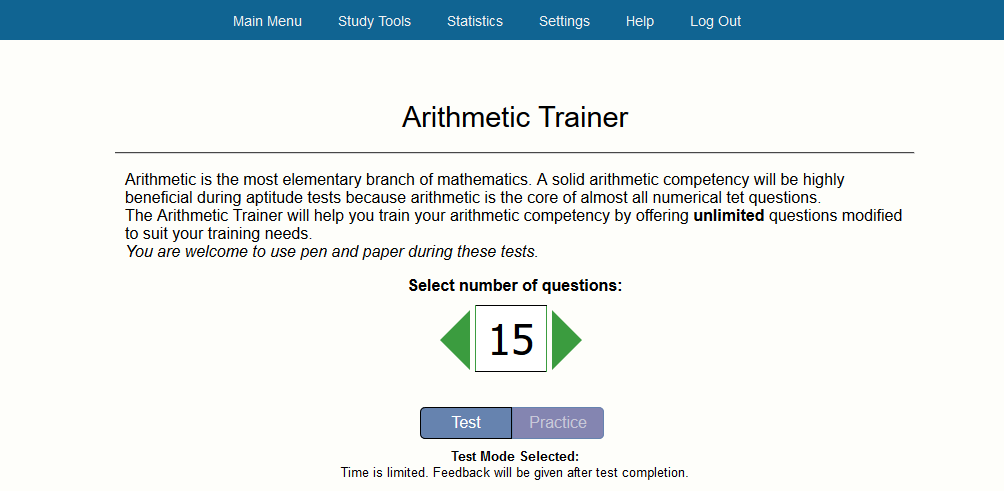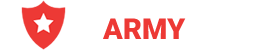Select Page

Mathematical Study Guide

Study for the Mathematical Knowledge sub-test of the ASVAB, for the Mathematical Ability test of the ADF YOU Session or for the mathematical questions of the CFAT. Theory cover up to 16 different subjects, every subject is followed by one or more questions to make sure you not only read the text but learn the theory.
N

N

N

N

N

N

N

Use the questions to determine if you know the theory and can skip the subject